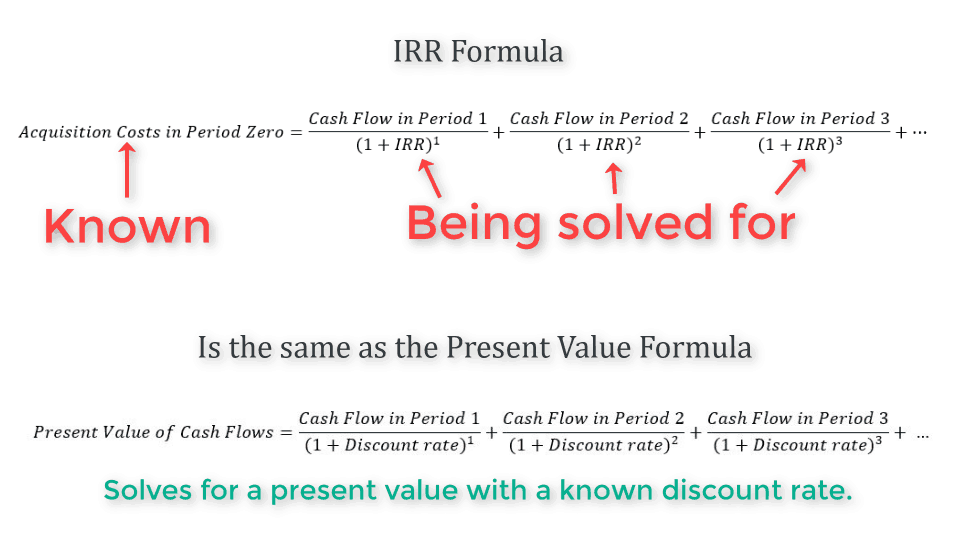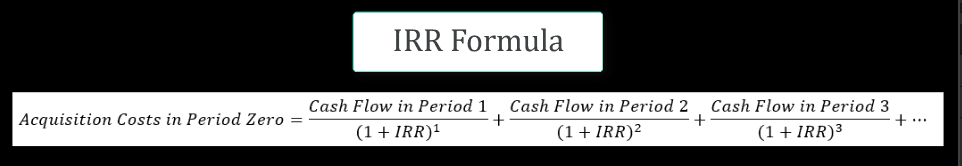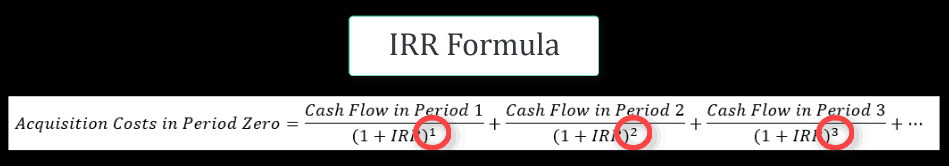# Deep Dive: The IRR and XIRR – Fully and Simply Explained

For my next post, I thought I would bring it back to some fundamentals and review the IRR and XIRR return metrics and functions in Excel. These are some of the most commonly used functions and return metrics in commercial real estate and they can be a bit complex to understand for those of us just starting out in real estate finance. The below video and slightly altered video transcription I hope will be a helpful guide and/or refresher.

Are you an Accelerator member? Check out the IRR vs. XIRR discussion, especially as it relates to LP and GP considerations in a monthly equity waterfall here and here. Not yet an Accelerator member? Consider joining the real estate financial modeling training program used by top real estate companies and elite universities to train the next generation of CRE professionals.

## Video – IRR Fully and Simply Explained

#### Loose transcription of the IRR Fully and Simply Explained Video

In this video, we dive into the IRR and XIRR functions in Excel. So to give a high level overview, The IRR and XIRR are important functions used frequently in real estate financial modeling and it’s used to calculate the Internal Rate of Return over an investment hold period. We can think of the Internal Rate of Return as the project’s self-derived discount rate.

Note: If the term discount rate is unfamiliar to you, then I’d suggest to first read my post and watch the video on valuing real estate using a discounted cash flow model

## When to Use IRR and When to Use XIRR

The traditional IRR function does the job for calculating the internal rate of return over yearly periods, but often times we find ourselves modeling over monthly periods and even quarterly periods to which using the IRR function would be incorrect and therefore we need to utilize XIRR, which again we will cover later in this post.

#### The Internal Rate of Return Formula

Before we get to the excel sheet, let’s do a quick review of the internal rate of return formula, which is really just the present value formula but rather than deriving a present value with a known discount rate, the present value is known and the discount rate, or IRR, in the formula is what needs to be solved for.Excel will iterate through this formula numerous times until the internal rate of return is solved for.## Using the XIRR Function in Real Estate

As previously stated, IRR and XIRR are some of the most commonly used functions and return metrics in commercial real estate and they can be a bit complex to understand for those just starting out in real estate finance. The below video and slightly altered video transcription I hope will be a helpful guide and/or refresher.

## Video – XIRR Fully and Simply Explained

Follow along as I explain the functionality of the XIRR() function in Excel.

## Review: The IRR Formula

Reminder: The IRR Formula is the Present Value Formula only instead of solving for the present value, we know the present value and we are backsolving for the discount rate (the IRR).As we said previously, the Present Value Formula can only be used if periods are in one-year increments, so using it for any other periodic payout or earning schedules would be incorrect.  So we need to alter this formula to account for payment or earning schedules that are different than one year periods.

## Transforming The IRR Formula to The XIRR Formula

So the question is, how do we alter the formula so that when there are payouts at different timing intervals other than a year it can properly solve for the internal rate of return? The answer is that we alter the exponent, which in the normal IRR formula is always commensurate with the year in the referenced period and dictates how the cash is being discounted back.We alter the exponent to a fraction with the numerator being the total days between the start date, or time period 0, and the current date. Then, for the denominator we use 365, or a year represented in days.By changing the unit of measure in the denominator of the exponent to a year represented in days allows us to now analyze any type of earnings or payout periods that are irregular as long as they are not split into anything smaller than a day.

Tags: